# Deep Neural Networks from scratch in Python

Source: Deep Learning on Medium

In this guide we will build a deep neural network, with as many layers as you want! The network can be applied to supervised learning problem with binary classification.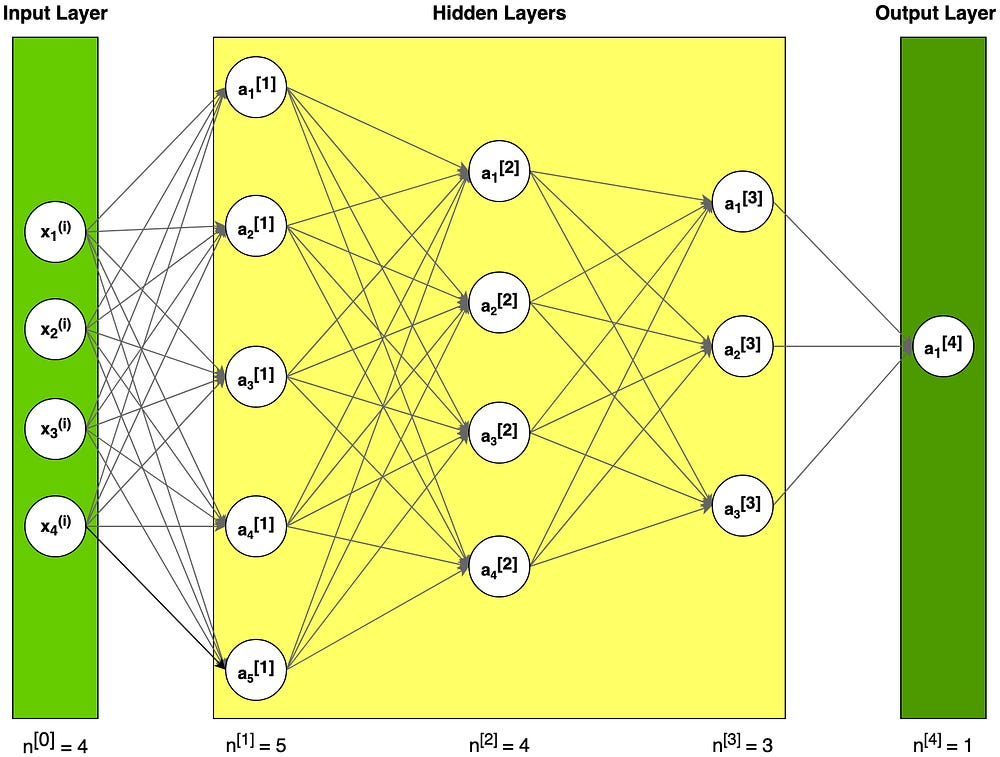Figure 1. Example of neural network architecture

#### Notation

Superscript [l] denotes a quantity associated with the lᵗʰ layer.

Superscript (i) denotes a quantity associated with the iᵗʰ example.

Lowerscript i denotes the iᵗʰ entry of a vector.

This article was written assuming that the reader is already familiar with the concept of a neural network. Otherwise, I recommend to read this nice introduction https://towardsdatascience.com/how-to-build-your-own-neural-network-from-scratch-in-python-68998a08e4f6

#### Single neuron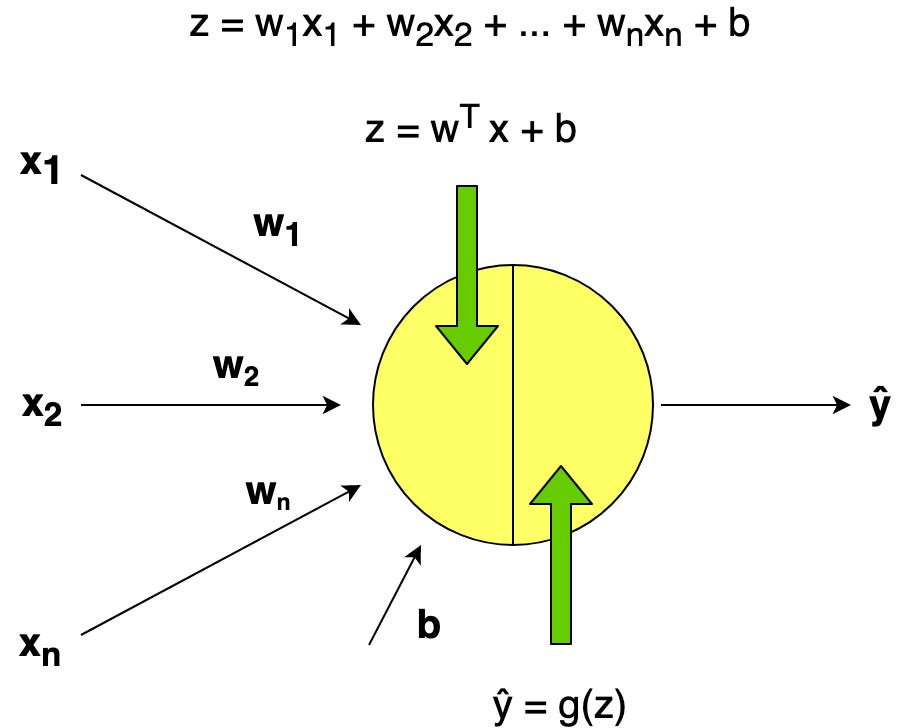Figure 2. Example of single neuron representation

A neuron computes a linear function (z = Wx + b) followed by an activation function. We generally say that the output of a neuron is a = g(Wx + b) where g is the activation function (sigmoid, tanh, ReLU, …).

#### Dataset

Let’s assume that we have a very big dataset with weather data such as temperature, humidity, atmospheric pressure and the probability of rain.

Problem statement:

• a training set of m_train weather data labeled as rain (1) or not (0)
• a test set of m_test weather data labeled as rain or not
• each weather data consists of x1 = temperature, x2 = humidity, x3 = atmospheric pressure

One common preprocessing step in machine learning is to center and standardize your dataset, meaning that you subtract the mean of the whole numpy array from each example, and then divide each example by the standard deviation of the whole numpy array.

### General methodology (building the parts of our algorithm)

We will follow the Deep Learning methodology to build the model:

1. Define the model structure (such as number of input features)
2. Initialize parameters and define hyperparameters:
• number of iterations
• number of layers L in the neural network
• size of the hidden layers
• learning rate α

3. Loop for num_iterations:

• Forward propagation (calculate current loss)
• Compute cost function
• Backward propagation (calculate current gradient)
• Update parameters (using parameters, and grads from backprop)

4. Use trained parameters to predict labels

#### Initialization

The initialization for a deeper L-layered neural network is more complicated because there are many more weight matrices and bias vectors. I provide the tables below in order to help you keep the right dimensions of the structures.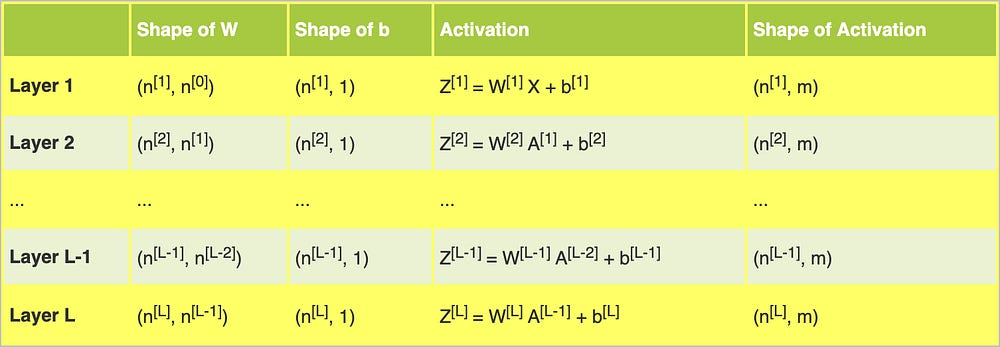Table 1. Dimensions of weight matrix W, bias vector b and activation Z for layer l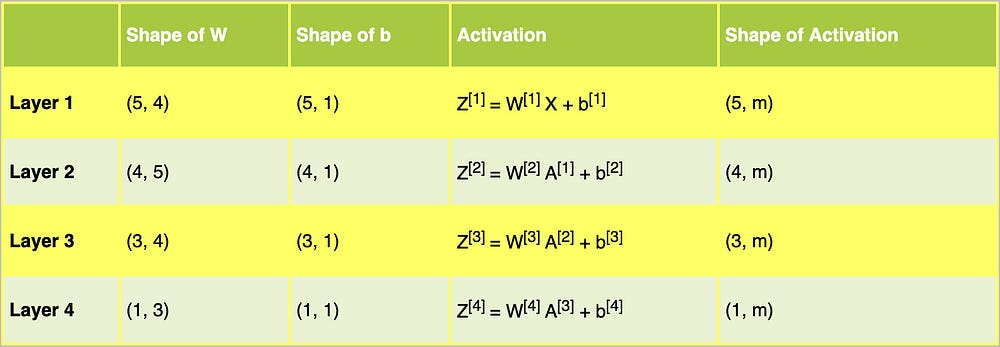Table 2. Dimensions of weight matrix W, bias vector b and activation Z for the neural network for our example architecture

Table 2 helps us prepare correct dimensions for the matrices of our example neural network architecture from Figure 1.Snippet 1. Initialization of the parameters

Parameters initialization using small random numbers is simple approach, but it guarantees good enough starting point for our algorithm.

Remember:

• Different initialization techniques such as Zero, Random, He or Xavier lead to different result
• Random initialization makes sure different hidden units can learn different things (initializing all the weights to zero causes, that every neuron in each layer will learn the same thing)
• Don’t initialize to values that are too large

#### Activation functions

Activation functions give the neural networks non-linearity. In our example, we will use sigmoid and ReLU.

Sigmoid outputs a value between 0 and 1 which makes it a very good choice for binary classification. You can classify the output as 0 if it is less than 0.5 and classify it as 1 if the output is more than 0.5.Snippet 2. Sigmoid and ReLU activation functions and their derivatives

In Snippet 2 you can see the vectorized implementation of activation functions and their derivatives (https://en.wikipedia.org/wiki/Derivative). The code will be used in the further calculation.

#### Forward propagation

During forward propagation, in the forward function for a layer l you need to know what the activation function in a layer is (Sigmoid, tanh, ReLU, etc.). Given input signal from the previous layer, we compute Z and then apply selected activation function.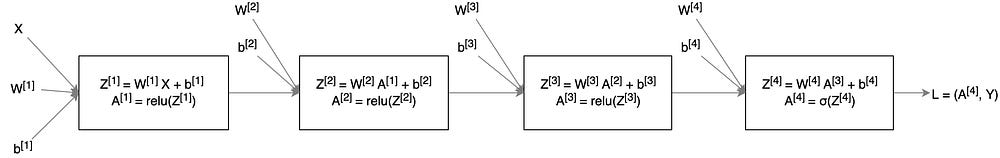Figure 3. Forward propagation for our example neural network

The linear forward module (vectorized over all the examples) computes the following equations:Equation 1. Linear forward functionSnippet 3. Forward propagation module

We use “cache” (Python dictionary, which contains A and Z values computed for particular layers) to pass variables computed during forward propagation to the corresponding backward propagation step. It contains useful values for backward propagation to compute derivatives.

#### Loss function

In order to monitor the learning process, we need to calculate the value of the cost function. We will use the below formula to calculate the cost.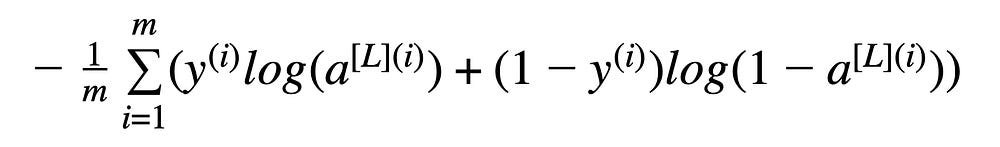Equation 2. Cross-entropy costSnippet 4. Computation of the cost function

#### Backward propagation

Backpropagation is used to calculate the gradient of the loss function with respect to the parameters. This algorithm is the recursive use of a “chain rule” known from differential calculus.

Equations used in backpropagation calculation: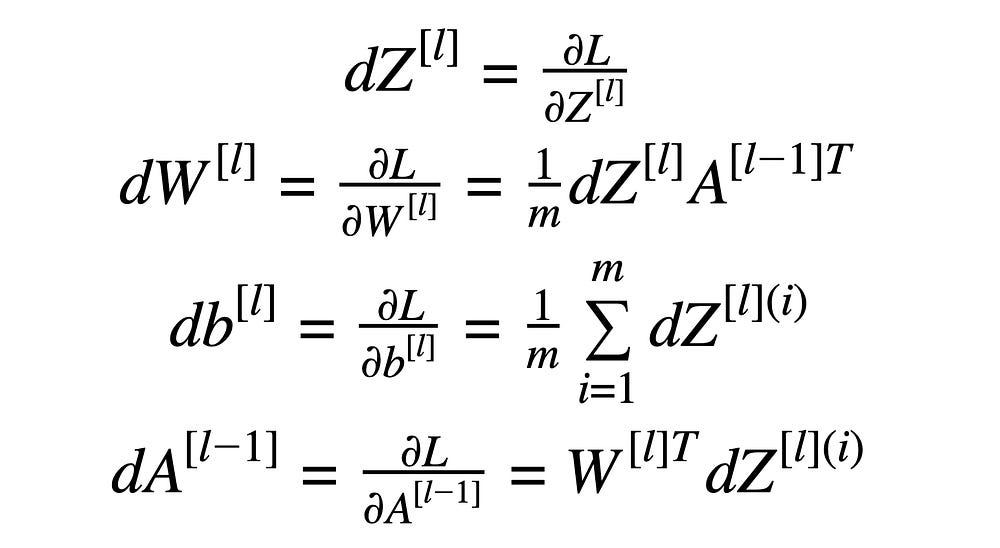Equation 3. Formulas for backward propagation calculation

The chain rule is a formula for calculating the derivatives of composite functions. Composite functions are functions composed of functions inside other function.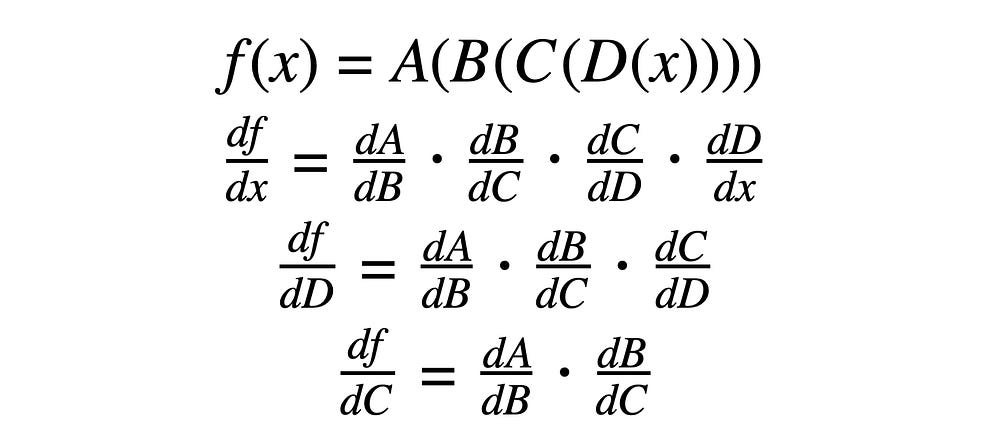Equation 4. Chain rule examples

It is difficult to calculate the loss without “chain rule” (equation 5 as an example).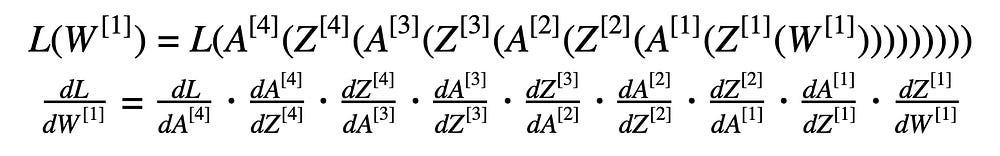Equation 5. Loss function (with substituted data) and its derivative with respect to the first weight.

The first step in backpropagation for our neural network model is to calculate the derivative of our loss function with respect to Z from the last layer. Equation 6 consists of two components, the derivative of the loss function from equation 2 (with respect to the activation function) and the derivative of the activation function “sigmoid” with respect to Z from the last layer.Equation 6. The derivative of the loss function with respect to Z from 4ᵗʰ layer

The result from equation 6 can be used to calculate the derivatives from equation 3:Equation 7. The derivative of the loss function with respect to A from 3ᵗʰ layer

The derivative of the loss function with respect to the activation function from the third layer (equation 7) is used in the further calculation.Equation 8. The derivatives for the third layer

The result from equation 7 and the derivative of the activation function “ReLU” from the third layer is used to calculate the derivatives from equation 8 (the derivative of the loss function with respect to Z). Following this, we make a calculation for equation 3.

We make similar calculations for equation 9 and 10.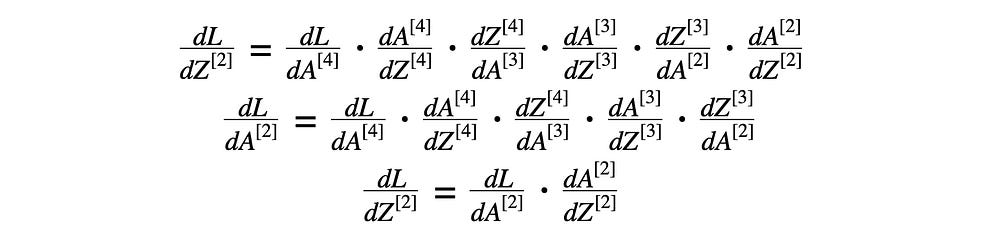Equation 9. The derivatives for the second layer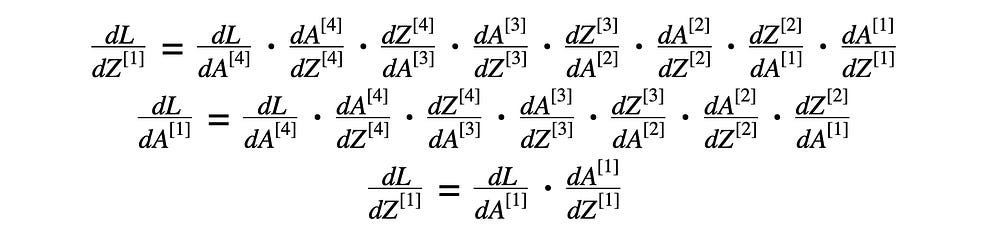Equation 10. The derivatives for the first layer

The general idea:

The derivative of the loss function with respect to Z from lᵗʰ layer helps to calculate the derivative of the loss function with respect to A from (l-1)ᵗʰ layer (the previous layer). Then the result is used with the derivative of the activation function.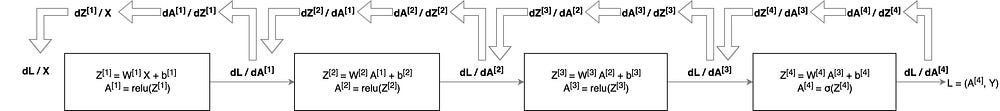Figure 4. Backward propagation for our example neural networkSnippet 5. Backward propagation module

#### Update parameters

The goal of the function is to update the parameters of the model using gradient optimization.Snippet 6. Updating parameters values using gradient descent

#### Full model

The full implementation of the neural network model consists of the methods provided in snippets.Snippet 7. The full model of the neural network

In order to make a prediction, you only need to run a full forward propagation using the received weight matrix and a set of test data.

You can modify nn_architecture in Snippet 1 to build a neural network with a different number of layers and sizes of the hidden layers. Moreover, prepare the correct implementation of the activation functions and their derivatives (Snippet 2). The implemented functions can be used to modify linear_activation_forward method in Snippet 3 and linear_activation_backward method in Snippet 5.

### Further improvements

You can face the “overfitting” problem if the training dataset is not big enough. It means that the learned network doesn’t generalize to new examples that it has never seen. You can use regularization methods such as L2 regularization (it consists of appropriately modifying your
cost function) or dropout ( it randomly shuts down some neurons in each iteration).

We used Gradient Descent to update the parameters and minimize the cost. You can learn more advanced optimization methods that can speed up learning and even get you to a better final value for the cost function for example:

• Mini-batch gradient descent
• Momentum
• Adam optimizer